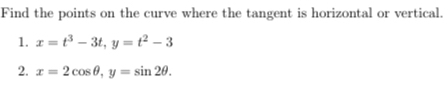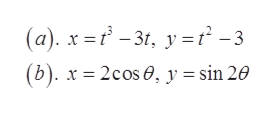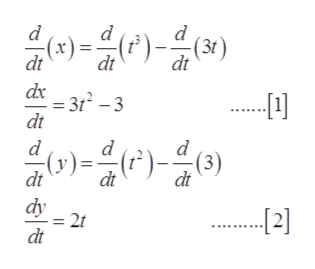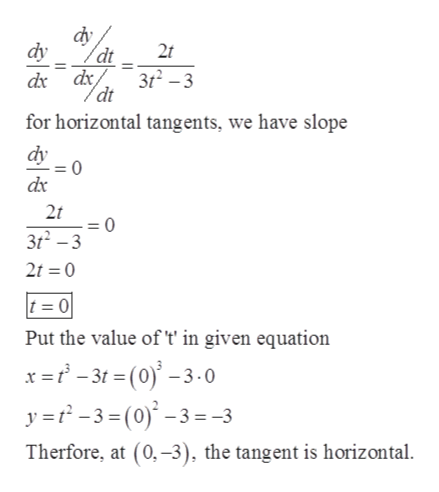# Find the points on the curve where the tangent is horizontal or vertical.1. 3t, y t2 -32. 2cos0, y= sin 20.

Question
38 viewshelp_outlineImage TranscriptioncloseFind the points on the curve where the tangent is horizontal or vertical. 1. 3t, y t2 -3 2. 2cos0, y= sin 20. fullscreen
check_circle

Step 1

Given:help_outlineImage Transcriptionclose(a). x -3t y = t2 -3 (b). x 2cos, y = sin 20 fullscreen
Step 2

(a). Differentiate ‘x’ and ‘y’ with respect to ‘t’:help_outlineImage Transcriptionclosed )-)() (1) dt (x) dt 3r2 -3 dt dt dx 음아올마음이 d d -(y) dt )=)() (3) dt dt dy = 2 dt fullscreen
Step 3

Now, find dy/dx by  and  and find the...help_outlineImage Transcriptionclose2t dx dx3t2 -3 dt for horizontal tangents, we have slope dy 2t 0 3t2-3 2t 0 t = 0 Put the value of 't in given equation x =-3t= (0)'-3-0 y =-3= (0)-3 =-3 Therfore, at (0,-3), the tangent is horizontal. fullscreen

### Want to see the full answer?

See Solution

#### Want to see this answer and more?

Solutions are written by subject experts who are available 24/7. Questions are typically answered within 1 hour.*

See Solution
*Response times may vary by subject and question.
Tagged in

### Derivative